# 1. Show if the following field is a Maxwellian equation (If it satisfy the 1st and...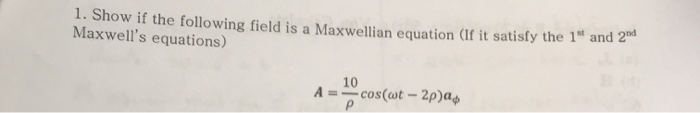1. Show if the following field is a Maxwellian equation (If it satisfy the 1st and 200 Maxwell's equations) 10 A = cos(wt - 2pa р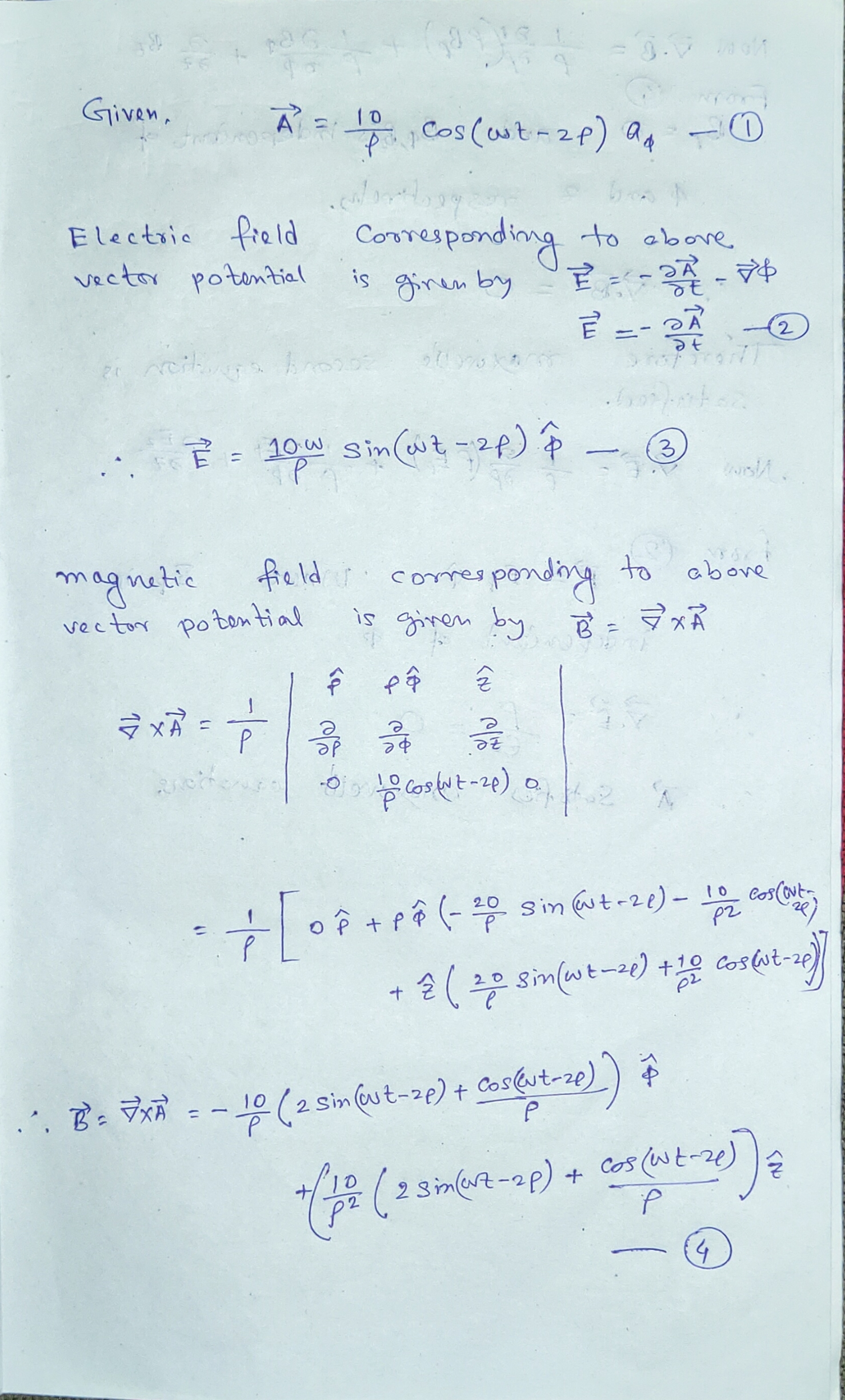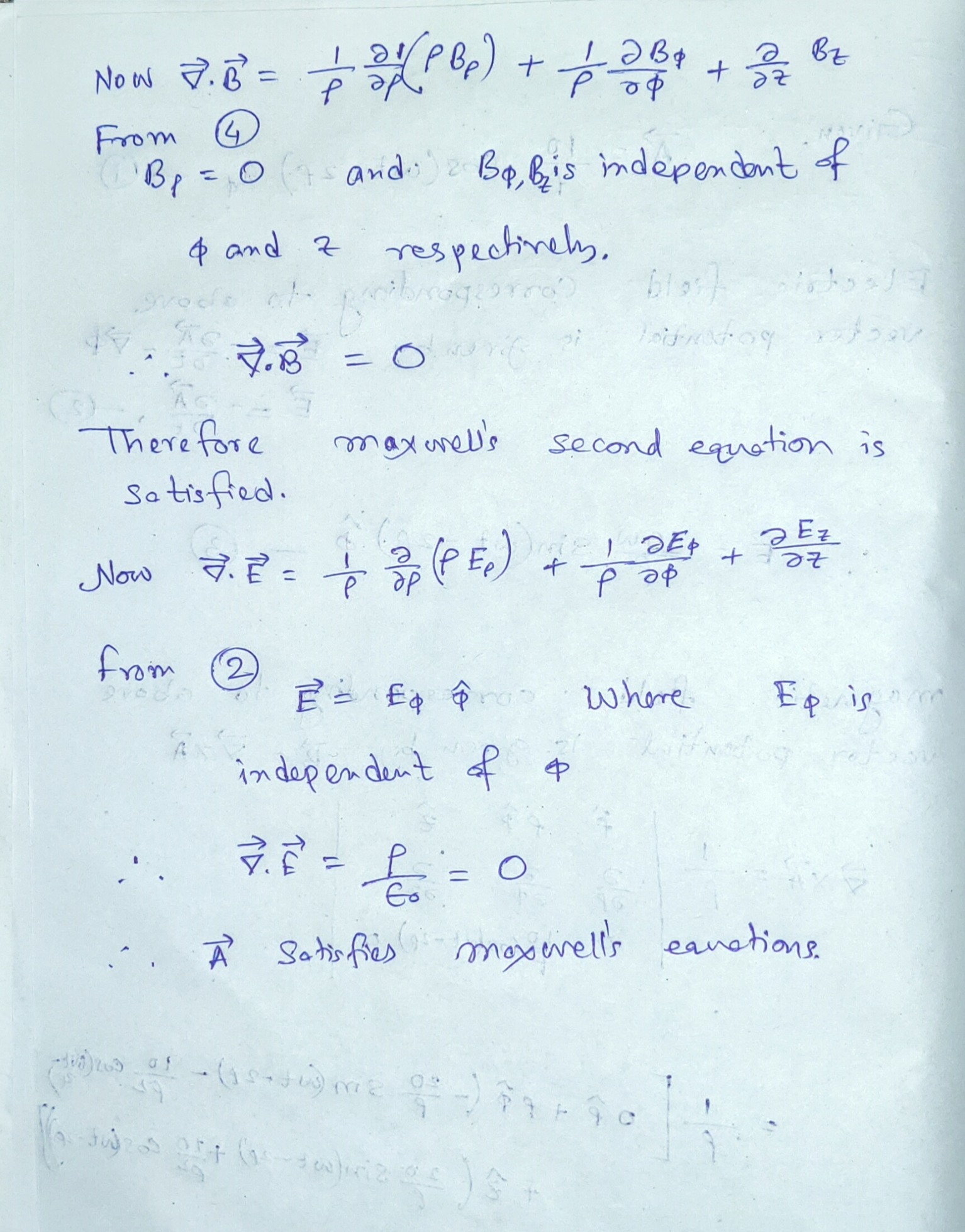##### Add Answer of: 1. Show if the following field is a Maxwellian equation (If it satisfy the 1st and...
Similar Homework Help Questions
• ### (a) Show that this field can satisfy Maxwell's equations if w and k are related in...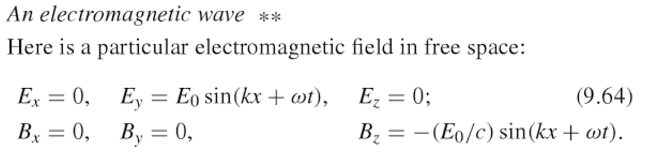(a) Show that this field can satisfy Maxwell's equations if w and k are related in a certain way. (b) Suppose w=1010s-1 and E0=1kV/m. What is the wavelength? What is the energy density in joules per cubic meter, averaged over a large region? From this calculate the power density, the energy flow in joules per square meter per second. (c) Show also that the electric field of associated with a spherically symmetric wave may have the dependence Ei = {Acos[k(r...

• ### (a) Show that this field can satisfy Maxwell's equations if w and k are related in...(a) Show that this field can satisfy Maxwell's equations if w and k are related in a certain way. (b) Suppose w=1010s-1 and E0=1kV/m. What is the wavelength? What is the energy density in joules per cubic meter, averaged over a large region? From this calculate the power density, the energy flow in joules per square meter per second. (c) Show also that the electric field of associated with a spherically symmetric wave may have the dependence Ei = {Acos[k(r...

• ### Wave Equation

Show that the following wave function satisfy the wave equationa) y(x,t)=A cos(kx+wt)b) y(x,t)=A sin(kx+wt)c) In what direction are these waves travelling?How can you tell?d) For the wave of parte) write the equations for the transverse velocity and transverse acceleration of a particle at point x.

• ### Stuck at this point in my E&M Wave problem"Suppose that in the absence of any matter and charges, an electric field exists of the form:E = E0cos(kz - wt) + E0 sin(kz - wt)Where E0cos(kz-wt) is in the x^ direction and E0sin(kz - wt) is in the y^ directionShow directly that E and B satisfy maxwell's equations and describe what they look like at the origin as a function of time. "I derived the magnetic field using the identity t = So I HaveB = (Eo * k/w)...

• ### Question 1: As you work through the parts of this question you are going to show...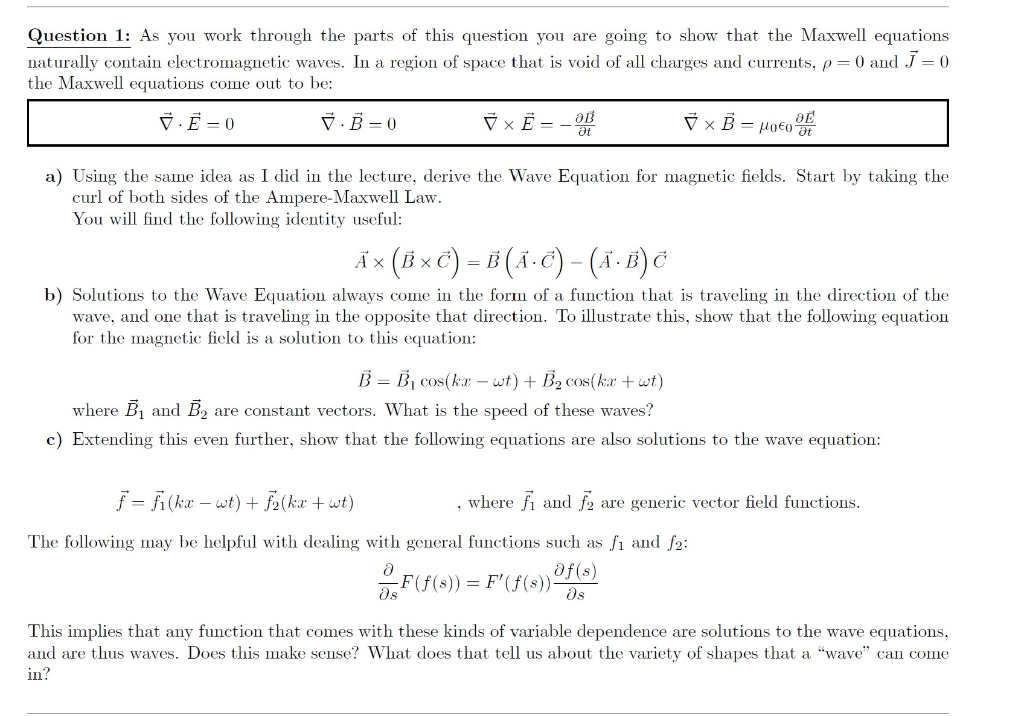Question 1: As you work through the parts of this question you are going to show that the Maxwell equations naturally contain electromagnetic waves. In a region of space that is void of all charges and currents, p=0 and J = 0 the Maxwell equations come out to be: Y E = 0 7.B=0 7 x Ē = -1 1 x B = Poco a) Using the same idea as I did in the lecture, derive the Wave Equation for...

• ### Show that the electric field: E(x,t)-E cos(kr-at)+E, cos(kx-of) satisfies Maxwell's equation: Here, ø, and oj are...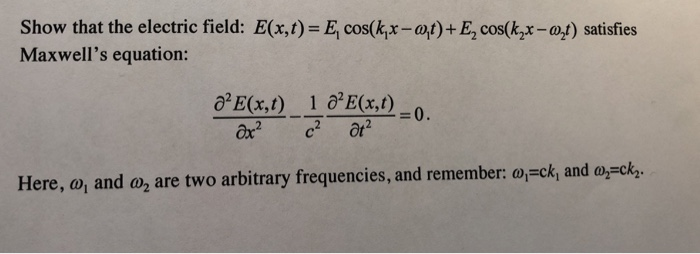Show that the electric field: E(x,t)-E cos(kr-at)+E, cos(kx-of) satisfies Maxwell's equation: Here, ø, and oj are two arbitrary frequencies, and remember oj-ck, and oj-ck quencies, and remember: ai-ck, and arch.

• ### 1. (5 points) This course deals with Maxwell's equation. When there is no chargeor current, the...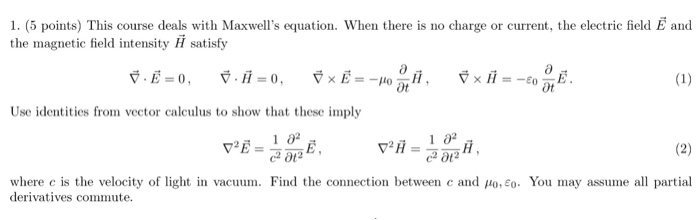1. (5 points) This course deals with Maxwell's equation. When there is no chargeor current, the electric field E and the magnetic field intensity satisfy Use identities from vector calculus to show that these imply เอ2 1 02 where c is the velocity of light in vacuum. Find the connection between cand ,10, eo. You may assume all partial derivatives commute

• ### PrOBleM: SoLuTiONS To THE WAvE EQuATION a) By direct substitution determine which of the following functions...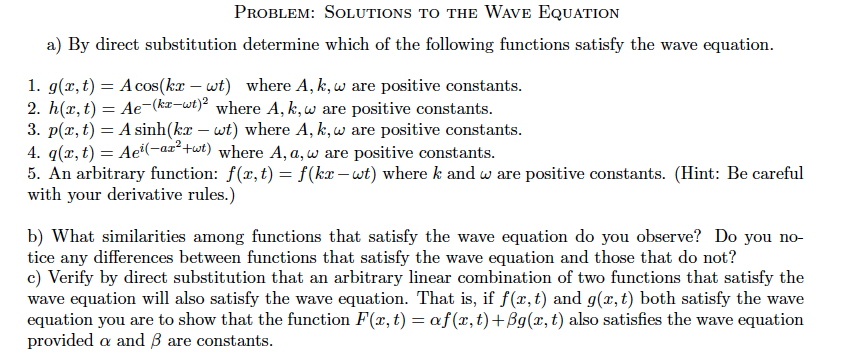PrOBleM: SoLuTiONS To THE WAvE EQuATION a) By direct substitution determine which of the following functions satisfy the wave equation 1. g(z, t)-A cos(kr - wt) where A, k, w are positive constants 2. h(z,t)-Ae-(kz-wt)2 where A, k, ω are positive constants 3. p(x, t) A sinh(kx-wt) where A, k,w are positive constants 4. q(z, t) - Ae(atut) where A,a, w are positive constants 5. An arbitrary function: f(x, t) - f(kx -wt) where k and w are positive constants....

• ### #4 3. Demonstrate that the following equations satisfy the "wave equation." For those that do, find the wa...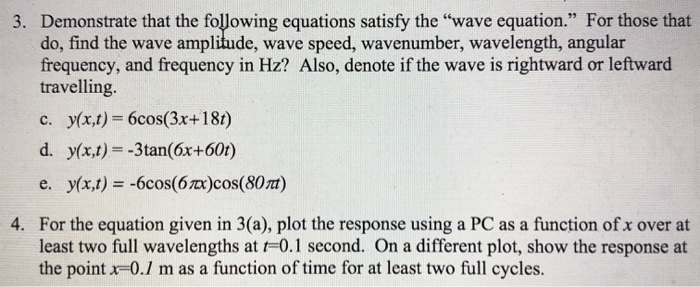#4 3. Demonstrate that the following equations satisfy the "wave equation." For those that do, find the wave amplitude, wave speed, wavenumber, wavelength, angular frequency, and frequency in Hz? Also, denote if the wave is rightward or leftward travelling. c. y(x,t) 6cos(3x+18t) d. y(x,t) -3tan(6x+60t) yx,t) -6cos(67x)cos(80t) e. 4. For the equation given in 3(a), plot the response using a PC as a function of x over at least two full wavelengths at r-0.1 second. On a different plot, show...

• ### As you work through the parts of this question you are going to show that the...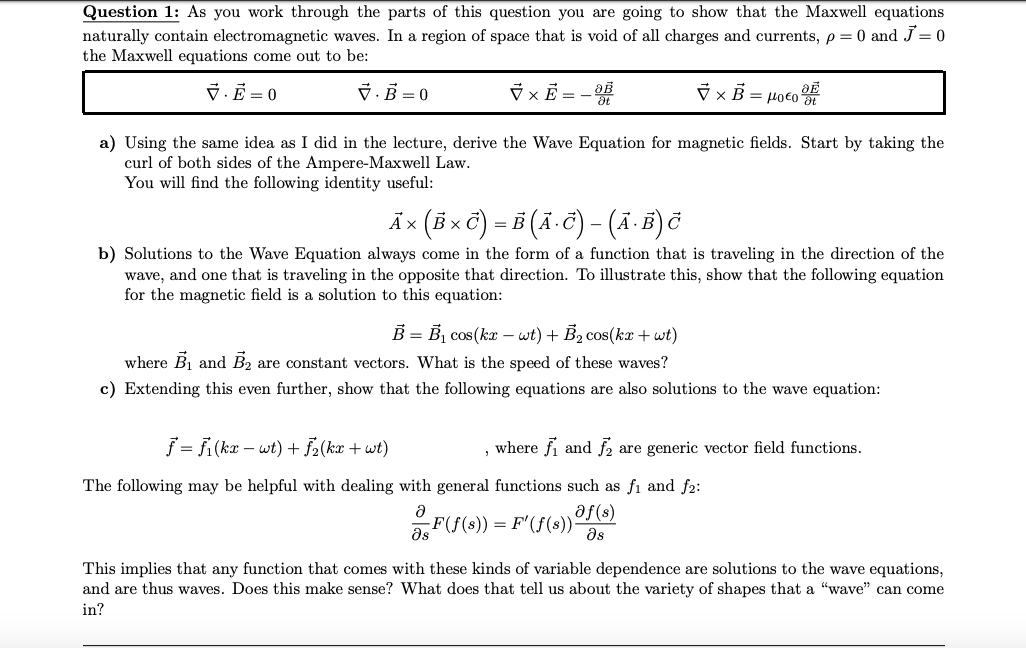As you work through the parts of this question you are going to show that the Maxwell equations naturally contain electromagnetic waves. In a region of space that is void of all charges and currents, ρ = 0 and J~ = 0 the Maxwell equations come out to be: Question 1: As you work through the parts of this question you are going to show that the Maxwell equations naturally contain electromagnetic waves. In a region of space that is...

Free Homework App